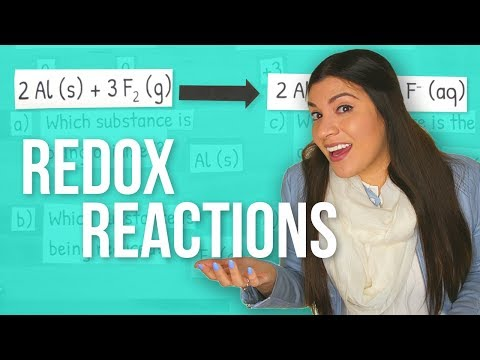# can anyone teach me how to do redox (equations) step by step? i dont get any of it. 10th grade chemistry?

Relevance

•Login to reply the answers
• Some discussion and lots of solved examples:

https://www.chemteam.info/Redox/Redox.html

•Login to reply the answers
• The Classic Example is iron(Fe) and potassium dichromate. in acidified solution.

Taking the dichromate half equation first

Cr2O7^2- Remember oxygen is always '-2' oxidation state.

Hence"cr + 7(-2) = -2

2Cr - 14 = -2

2Cr = 12

Cr = +6 (oxidation state) this is reduced to (+3)

Hence Cr2O7^2- + 14H^+ 6e^- = 2Cr^3+ 7H2O

Notice the Cr is now 2Cr^3+

The 7 oxygens become 7 water

We 'back' calculate the hydrogens from the water as '14'

Then we balance the charges

-2 + 14 -(x)e = 2(+3)

-2 + 14 - xe^- = +6

xe^- = +6 - 14 + 2 = -6

So the 'x' of electrons is '6'

So the half equation is

Cr2O7^2- + 14H^+ 6e^- = 7H2O + 2Cr^3+ is the reductant side.

This will oxidise Iron(II) to Iron)III)

The half equation for iron is

Fe^2+ = Fe^3+ + e^-

We notice that there are 6 electrons in the dichromate half equation, so we need 6 e^- in the iron half equation.

Hence 6Fe^2+ = 6Fe^3+ + 6e^-

We now have two half equations viz.

Cr2O7^2- + 14H^+ 6e^- = 7H2O + 2Cr^3+

6Fe^2+ = 6Fe^3+ + 6e^-

We now add the two half equations, this will eliminate the electrons (e^-)

Cr2O7^2- + 14H^+ + 6Fe^2+ = 2Cr^3+ + 7H2O + 6Fe^3+

Notice that the elements/atoms balance, and more particularly the charges balance, at '+26' on both sides.

Hope that helps!!!!

•Login to reply the answers
• If you want step-by-step  .. Try Kahn Academy .. an online teaching site.  It could be a very detailed set of instructions ... Give a google search for Kahn Academy and the lesson you need .. Redox Reactions

•Login to reply the answers•Login to reply the answers